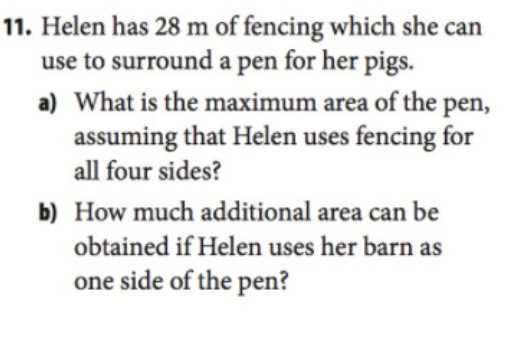### ¿Todavía tienes preguntas de matemáticas?

Pregunte a nuestros tutores expertos
Algebra
Pregunta11. Helen has $$28 m$$ of fencing which she can use to surround a pen for her pigs. a) What is the maximum area of the pen, assuming that Helen uses fencing for all four sides? b) How much additional area can be obtained if Helen uses her barn as one side of the pen?

$$A(x)= x(14- x)= -x^2+ 14x\\\Rightarrow A_{\max}= A(7)= 49m^2$$
$$A(x)= x(28- 2x)= -2x^2+ 28x\\\Rightarrow A_{\max}= A(7)= 98\\98- 49= 49m^2$$